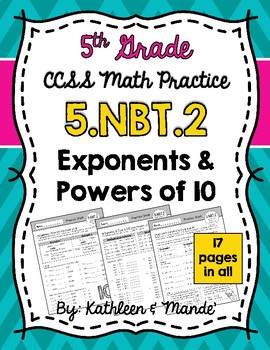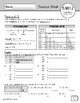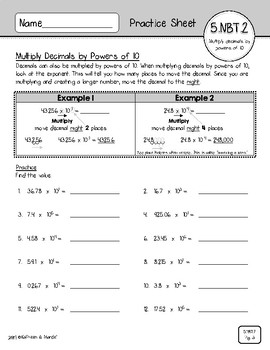# 5.NBT.2 Practice Sheets: Exponents & Powers of 10Subject
Resource Type
File Type

PDF

(2 MB|25 pages)
Standards
Also included in:
1. This CCSS aligned bundle has everything you need to teach 5.NBT.2: Multiply & Divide by Powers of 10. By purchasing the bundle, you can save 15%! (If purchased individually, this bundle would be \$11.25) Click each link below to see each product's detailed description and preview.This bundle i
\$11.25
\$9.60
Save \$1.65
• Product Description
• StandardsNEW

This 5.NBT.2 CCSS aligned place value packet includes 17 practice sheets to teach/reinforce multiplying and dividing whole numbers and decimals by powers of 10. These sheets can be used to introduce a concept, review a concept, or send home as reinforcement for homework. An answer key is provided.

------------------------------------------------------------------------------------------------------------

Check out our other 5.NBT.2 resources:

5.NBT.2 Task Cards: Multiply & Divide by Powers of 10

5.NBT.2 Matching Cards: Multiply & Divide by Powers of 10

5.NBT.2 Activity: Flip, Roll, Solve (Multiply & Divide by Powers of 10)

-------------------------------------------------------------------------------------------------------

Skills included in this packet:

- Page 1 Vocabulary & Powers of 10

- Page 2 Multiply Whole Numbers by Powers of 10

- Page 3 Multiply Decimals by Powers of 10

- Page 4 More Practice: Multiply Decimals by Powers of 10

- Page 5 Multiplication: Determine the Power of 10

- Page 6 Multiplication Patterns: Powers of 10

- Page 7 Mixed Practice: Multiply by Powers of 10

- Page 8 Divide Whole Numbers by Powers of 10

- Page 9 Divide Decimals by Powers of 10

- Page 10 More Practice: Divide Decimals by Powers of 10

- Page 11 Division: Determine the Power of 10

- Page 12 Division Patterns: Powers of 10

- Page 13 Mixed Practice: Divide by Powers of 10

- Page 14 Multiply & Divide by Powers of 10

- Page 15 More Practice: Multiply & Divide by Powers of 10

- Page 16 Multiplication & Division Patterns: Powers of 10

- Page 17 Multiple Choice: Powers of 10

Question formats include open response and multiple choice.

Enjoy! Teaching Together,

Kathleen & Mande'

Explain patterns in the number of zeros of the product when multiplying a number by powers of 10, and explain patterns in the placement of the decimal point when a decimal is multiplied or divided by a power of 10. Use whole-number exponents to denote powers of 10.
Total Pages
25 pages
Included
Teaching Duration
N/A
Report this Resource to TpT
Reported resources will be reviewed by our team. Report this resource to let us know if this resource violates TpT’s content guidelines.